Linear Regression and Correlation

70 Linear Equations

Linear regression for two variables is based on a linear equation with one independent variable. The equation has the form: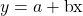where a and b are constant numbers.

The variable x is the independent variable, and y is the dependent variable. Another way to think about this equation is a statement of cause and effect. The X variable is the cause and the Y variable is the hypothesized effect. Typically, you choose a value to substitute for the independent variable and then solve for the dependent variable.

The following examples are linear equations.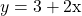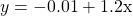The graph of a linear equation of the form y = a + bx is a straight line. Any line that is not vertical can be described by this equation.

Graph the equation y = –1 + 2x.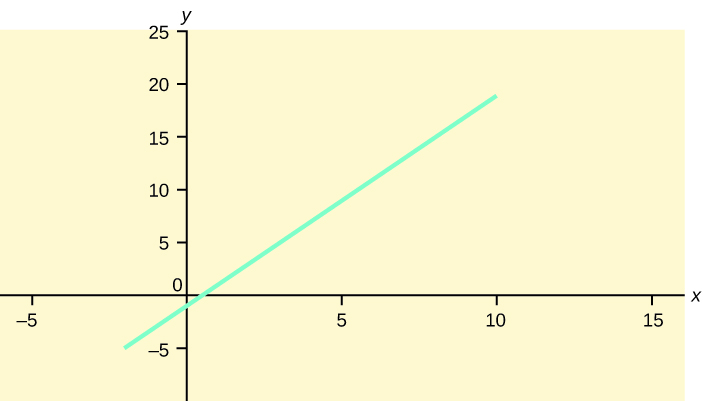Try It

Is the following an example of a linear equation? Why or why not?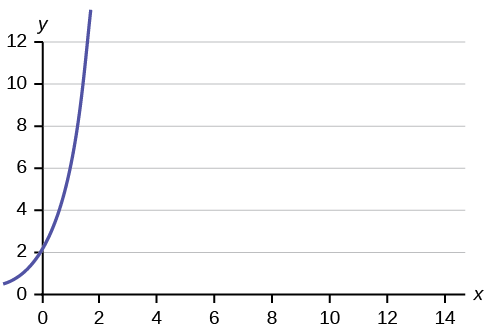No, the graph is not a straight line; therefore, it is not a linear equation.

Aaron’s Word Processing Service (AWPS) does word processing. The rate for services is ?32 per hour plus a ?31.50 one-time charge. The total cost to a customer depends on the number of hours it takes to complete the job.

Find the equation that expresses the total cost in terms of the number of hours required to complete the job.

Let x = the number of hours it takes to get the job done.
Let y = the total cost to the customer.

The ?31.50 is a fixed cost. If it takes x hours to complete the job, then (32)(x) is the cost of the word processing only. The total cost is: y = 31.50 + 32x

Slope and Y-Intercept of a Linear Equation

For the linear equation y = a + bx, b = slope and a = y-intercept. From algebra recall that the slope is a number that describes the steepness of a line, and the y-intercept is the y coordinate of the point (0, a) where the line crosses the y-axis. From calculus the slope is the first derivative of the function. For a linear function the slope is dy / dx = b where we can read the mathematical expression as “the change in y (dy) that results from a change in x (dx) = b * dx“.

Three possible graphs of y = a + bx. (a) If b > 0, the line slopes upward to the right. (b) If b = 0, the line is horizontal. (c) If b < 0, the line slopes downward to the right.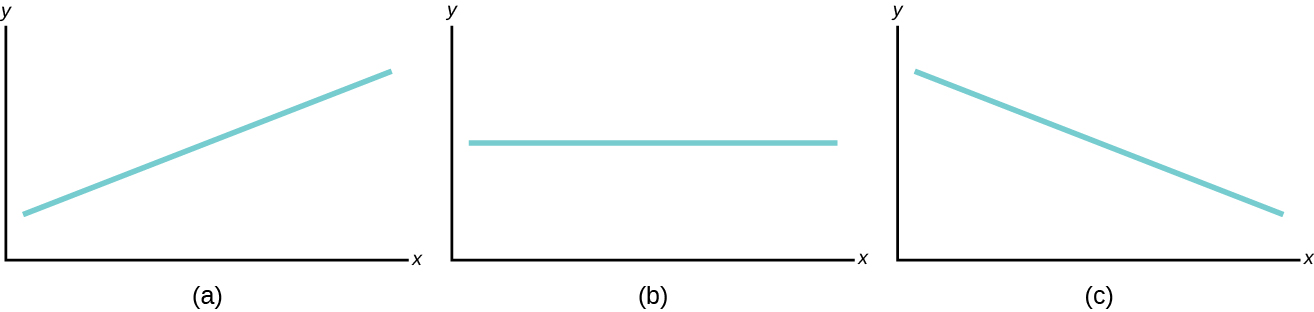Svetlana tutors to make extra money for college. For each tutoring session, she charges a one-time fee of ?25 plus ?15 per hour of tutoring. A linear equation that expresses the total amount of money Svetlana earns for each session she tutors is y = 25 + 15x.

What are the independent and dependent variables? What is the y-intercept and what is the slope? Interpret them using complete sentences.

The independent variable (x) is the number of hours Svetlana tutors each session. The dependent variable (y) is the amount, in dollars, Svetlana earns for each session.

The y-intercept is 25 (a = 25). At the start of the tutoring session, Svetlana charges a one-time fee of ?25 (this is when x = 0). The slope is 15 (b = 15). For each session, Svetlana earns ?15 for each hour she tutors.

True or False? If False, correct it: Suppose a 95% confidence interval for the slope β of the straight line regression of Y on X is given by -3.5 < β < -0.5. Then a two-sided test of the hypothesis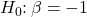would result in rejection of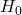at the 1% level of significance.

False. Sincewould not be rejected at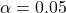, it would not be rejected at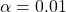.

True or False: It is safer to interpret correlation coefficients as measures of association rather than causation because of the possibility of spurious correlation.

True

We are interested in finding the linear relation between the number of widgets purchased at one time and the cost per widget. The following data has been obtained:

X: Number of widgets purchased – 1, 3, 6, 10, 15

Y: Cost per widget(in dollars) – 55, 52, 46, 32, 25

Suppose the regression line is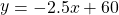. We compute the average price per widget if 30 are purchased and observe which of the following?

1.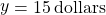; obviously, we are mistaken; the prediction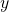is actually +15 dollars.
2., which seems reasonable judging by the data.
3.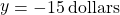, which is obvious nonsense. The regression line must be incorrect.
4., which is obvious nonsense. This reminds us that predicting Y outside the range of X values in our data is a very poor practice.

d

Discuss briefly the distinction between correlation and causality.

Some variables seem to be related, so that knowing one variable’s status allows us to predict the status of the other. This relationship can be measured and is called correlation. However, a high correlation between two variables in no way proves that a cause-and-effect relation exists between them. It is entirely possible that a third factor causes both variables to vary together.

True or False: If r is close to + or -1, we shall say there is a strong correlation, with the tacit understanding that we are referring to a linear relationship and nothing else.

True

Chapter Review

The most basic type of association is a linear association. This type of relationship can be defined algebraically by the equations used, numerically with actual or predicted data values, or graphically from a plotted curve. (Lines are classified as straight curves.) Algebraically, a linear equation typically takes the form y = mx + b, where m and b are constants, x is the independent variable, y is the dependent variable. In a statistical context, a linear equation is written in the form y = a + bx, where a and b are the constants. This form is used to help readers distinguish the statistical context from the algebraic context. In the equation y = a + bx, the constant b that multiplies the x variable (b is called a coefficient) is called as the slope. The slope describes the rate of change between the independent and dependent variables; in other words, the rate of change describes the change that occurs in the dependent variable as the independent variable is changed. In the equation y = a + bx, the constant a is called as the y-intercept. Graphically, the y-intercept is the y coordinate of the point where the graph of the line crosses the y axis. At this point x = 0.

The slope of a line is a value that describes the rate of change between the independent and dependent variables. The slope tells us how the dependent variable (y) changes for every one unit increase in the independent (x) variable, on average. The y-intercept is used to describe the dependent variable when the independent variable equals zero. Graphically, the slope is represented by three line types in elementary statistics.

Key Terms

Y – the dependent variable
Also, using the letter “y” represents actual values whilerepresents predicted or estimated values. Predicted values will come from plugging in observed “x” values into a linear model.
X – the independent variable
This will sometimes be referred to as the “predictor” variable, because these values were measured in order to determine what possible outcomes could be predicted.
a is the symbol for the Y-Intercept
Sometimes written as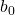, because when writing the theoretical linear model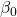is used to represent a coefficient for a population.
b is the symbol for Slope
The word coefficient will be used regularly for the slope, because it is a number that will always be next to the letter “x.” It will be written as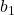when a sample is used, and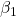will be used with a population or when writing the theoretical linear model.